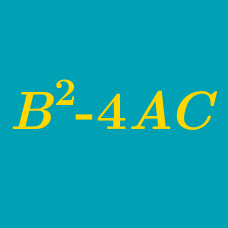Algebra

# Quadratic Discriminant - Repeated Roots

$ax^2 + 2bx + c = 0$

has 2 repeated roots, which of the following equations must be true?

If the graph of the quadratic function $f(x)= x^2 + 14x + a$ is tangent to the $x$-axis, what is $a?$

If the quadratic equation $f(x) = x^2-2(k-a)x+k^2+a^2-4k-b+15$ has a repeated root for all values of $k$, what is the value of $a+b\$?

How many integers $a$ are there such that at least one of the two quadratic equations $x^2-2ax+9=0, x^2-2ax+11a+12=0$ has complex, non-real roots?

If the quadratic $kx^2-16x+1$ is a perfect square polynomial, what is the value of $k$?

×